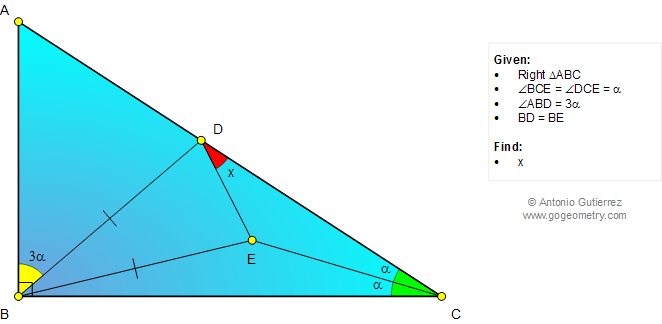# Math: Geometry Problem 1026: Right Triangle, 90 Degrees, Triple Angle, Congruence. Level: School, College.

< PREVIOUS PROBLEM  |  NEXT PROBLEM >

 The figure below shows a right triangle ABC (angle B = 90 degrees) with angle ABD = 3.angle BCE = 3.angle DCE, and BD = BE. Find the measure of angle CDE.Home | SearchGeometry | Problems | All Problems | Open Problems | Visual Index | 10 Problems | Problems Art Gallery Art | 1021-1030 | Triangle | Double Angle | Angle Bisector | Email | by Antonio Gutierrez

Add a solution to the problem 1026
Last updated: Sep 6, 2014